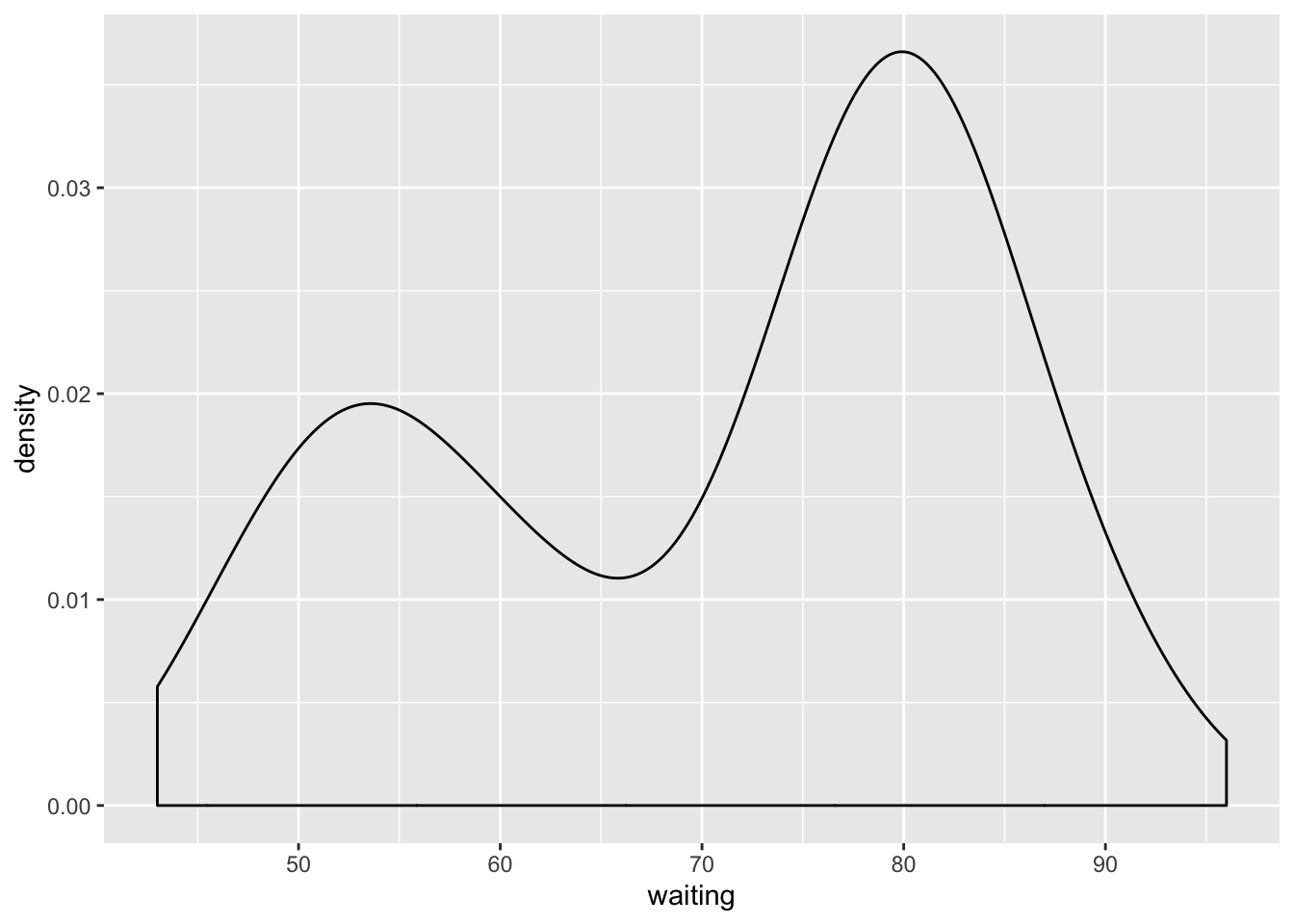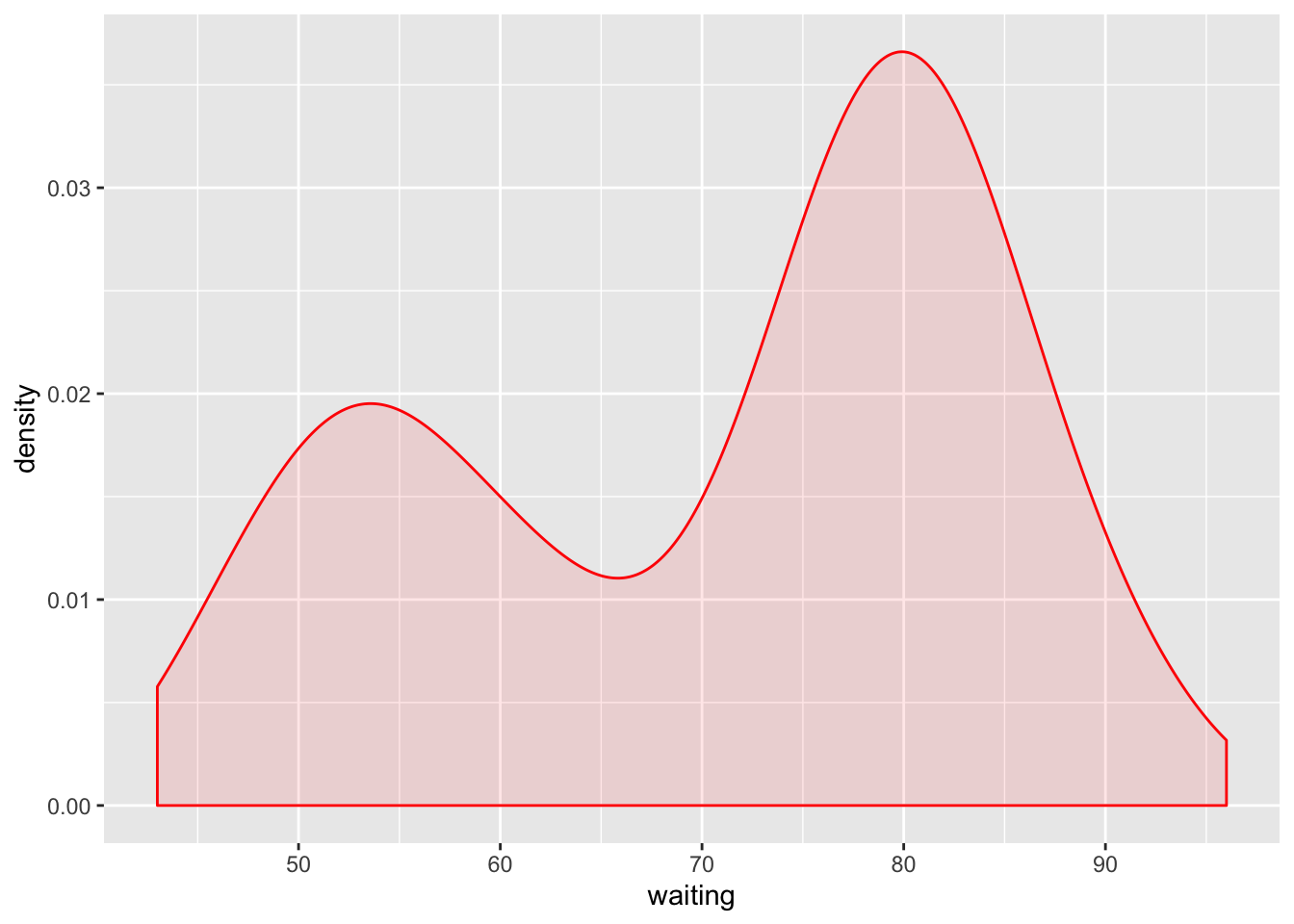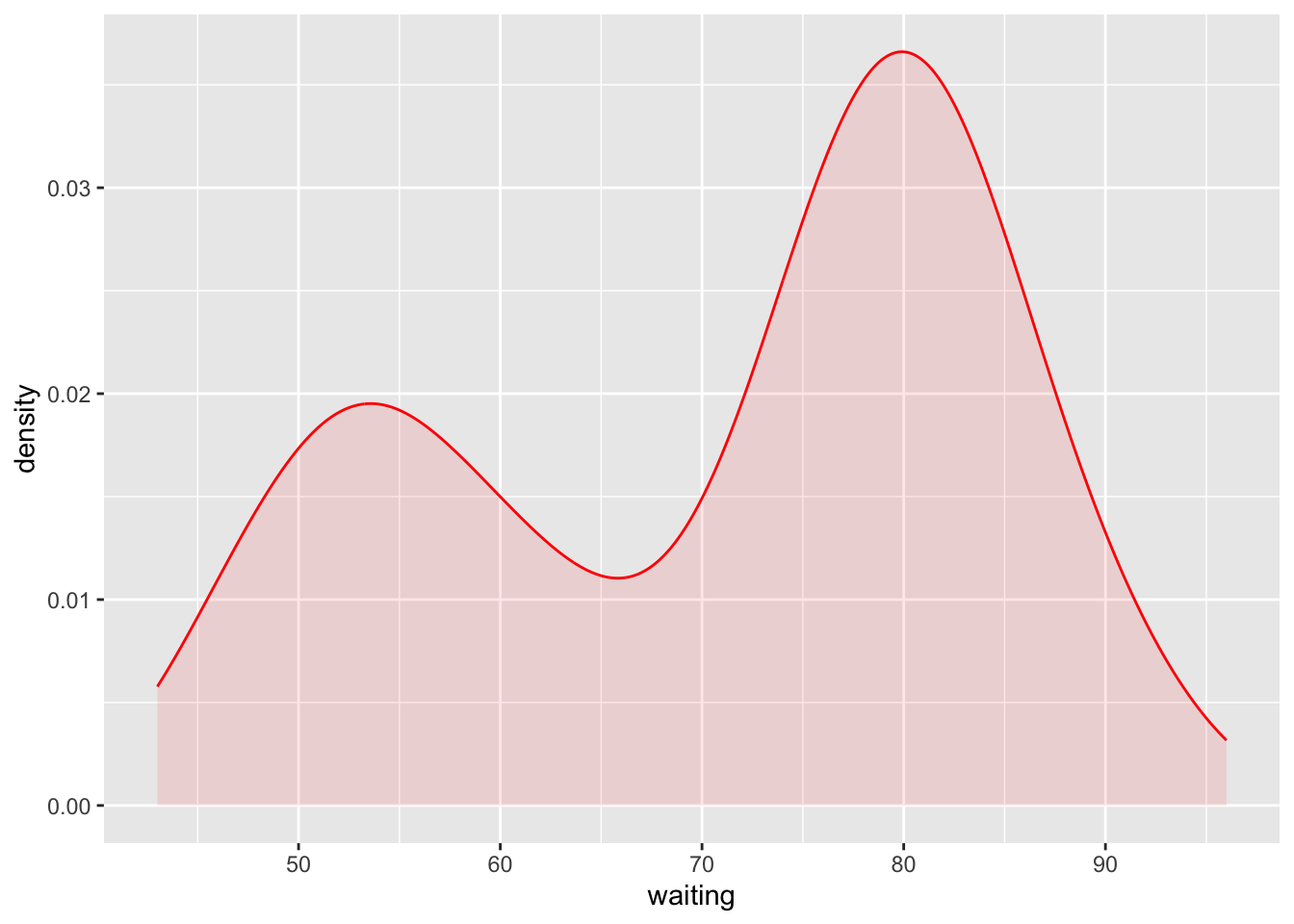# Statistics, Science, Random Ramblings

## A blog mostly about data and R

Visualising density of a variable is a common and useful step of exploring data. There is a `geom_density()` in ggplot2 that allows for straightforward visualisation of density, but without much effort the appearance of the plot can be improved.

Let’s use the `faithful` data. It contains the duration of eruptions of a geyser and the time between eruptions, both in minutes.

``````library("ggplot2")
library("tibble")
data("faithful")
faithful <- as_tibble(faithful)
faithful``````
``````## # A tibble: 272 x 2
##    eruptions waiting
##        <dbl>   <dbl>
##  1      3.6       79
##  2      1.8       54
##  3      3.33      74
##  4      2.28      62
##  5      4.53      85
##  6      2.88      55
##  7      4.7       88
##  8      3.6       85
##  9      1.95      51
## 10      4.35      85
## # … with 262 more rows``````

And now visualise the density of the `waiting` variable:

``````plot_base <- ggplot(faithful) + aes(x = waiting)
plot_base + geom_density()``````This gets the job done, but you might want to add some colour:

``plot_base + geom_density(fill = "red", alpha = .1, colour = "red")``To be honest, I am not really a fan of the connected line at the bottom and the side (although the vertical lines at the sides are technically correct).

By looking under the hood we can see how the density is calculated in ggplot2 and how it is plotted.

So, we can implement something similar:

``````dens <- density(faithful\$waiting, from = min(faithful\$waiting),
to = max(faithful\$waiting))
dens_tbl <- tibble(x = dens\$x, y = dens\$y)
ggplot(dens_tbl) +
aes(x = x, y = y) +
geom_area(fill = "red", alpha = .1) +
geom_line(colour = "red") +
labs(x = "waiting", y = "density")``````This could easily be put inside of a function or defined as a custom geom to use with `ggplot2`.

## Concluding remarks

Building an alternative to the default density plot of `ggplot2` is easy. Looking at the source of you favourite function can be useful and allows you to build upon what is included in a package.